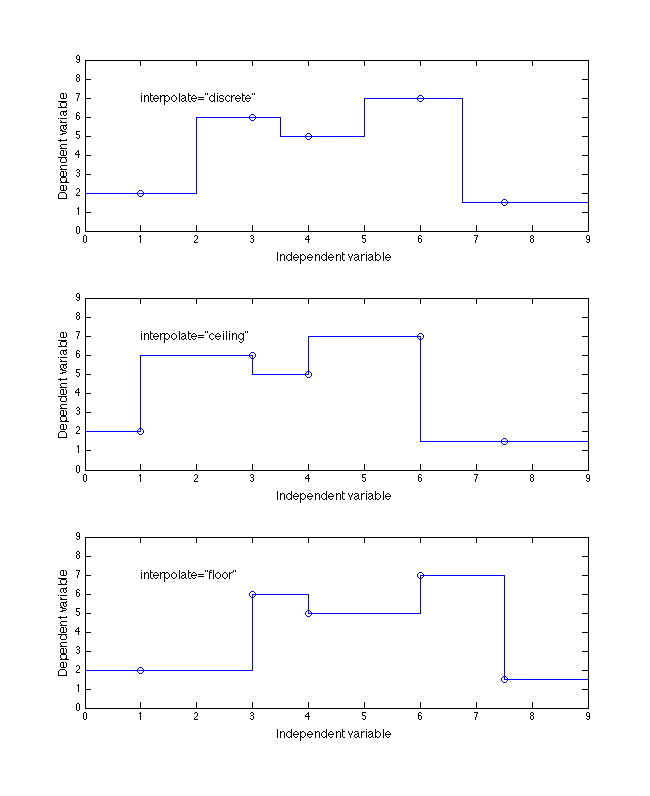### 6.3. Function interpolation/extrapolation

It is possible to specify the method of interpolation to be used for nonlinear function tables by use of the `interpolate` attribute of the `independentVarPts` and `independentVarRef` elements. This attribute, combined with the `extrapolate` flag, provides several different ways of realizing the intermediate values of the function when not at one of the specified intersections of independent values.

The following implementation notes are suggested:

• An infinite set of quadratic interpolations are possible; it is suggested to use the one that minimizes either the deviation from a linear interpolation or the slope error at any edge.

• For cubic interpolation, the natural cubic spline (which has a second derivative of zero at each end) is recommended when the `extrapolate` attribute is `none`. When the `extrapolate` attribute is `both`, a clamped cubic spline that matches the extrapolated slope of the last two data points is suggested.

• For the discrete interpolation values (`discrete`, `ceiling`, or `floor`), the value of the `extrapolate` attribute is meaningless.

For discrete interpolation,

• `discrete` implies the change between output values occurs midway between independent breakpoint values, as shown in the top plot of Figure 4.

• `ceiling` means the output takes on the value of the next-higher dependent variable breakpoint as soon as each independent breakpoint value is passed (assuming the input value is increasing) as shown in the middle plot of Figure 4.

• `floor` means the output retains the value of the last dependent variable breakpoint until the next independent breakpoint value is reached (assuming the input value is increasing) as shown in the bottom plot of Figure 4.

The default value for `interpolate` is `linear`. The default value for `extrapolate` is `neither`.

Figures 4 and 5 below give nine different examples for a 1D table whose independent values are [1, 3, 4, 6, 7.5] with dependent values of [2, 6, 5, 7, 1.5].

Figure 4.  Example of the three discrete enumeration values of `interpolate` attribute of the `independentVarPts` and `independentVarRef` elements for a 1D function table.2011-03-31